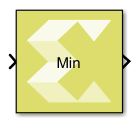# Min - 2022.1 English

## Vitis Model Composer User Guide (UG1483)

Document ID
UG1483
Release Date
2022-05-26
Version
2022.1 English

Outputs the minimum value of an input or element-wise minimum value of multiple inputs.

## Library

Math Functions/Math Operation## Description

The Min block with a single scalar or vector input outputs the minimum value of the input.

If the block has more than one input, the non-scalar inputs must have the same dimensions. Any scalar input is expanded to the dimensions of non-scalar inputs and the block outputs element-wise minimum value of the inputs.

## Data Type Support

• The block supports floating-point, integer, and fixed-point data types.
• The block supports real valued inputs.
• Number of inputs = 1.
• The block supports a scalar or vector (1-D or 2-D) input when it has only one input port. The output is a scalar.
• Number of inputs > 1.
• The block supports scalar, vector, or matrix inputs when it has more than one input port.
• The output has the same dimensions as those of the inputs when all the inputs have the same dimensions.
• The block supports mixed dimensions for inputs when it has more than one input port, provided all the non-scalar inputs have the same dimensions. Any scalar input is expanded to the dimensions of non-scalar inputs and the block outputs element-wise minimum value of the inputs. The output has the same dimensions as those of the non-scalar inputs.
• The block supports inputs that have different data types. The output data type in this case is defined by the following set of rules.
• If the data type of one of the inputs is a floating point type, the data type of the output is the floating point type among the data types of the inputs with the most precision.
• Otherwise, if the data type of one of the inputs is a fixed-point type, the data type of the output is the smallest fixed-point type capable of representing the result without any loss of precision.
• Otherwise, if the inputs are integral, the output is integral. If any input is signed, the output is signed. The bit width of the output is the largest among the bit widths of the inputs.

## Parameters

Number of inputs

This parameter determines the number of inputs.

Table 1. Number of Inputs
Choices Description
1 Initially, the block icon is created with a single input.
N

A positive integer value.

The block icon is redrawn with the specified number of input ports.